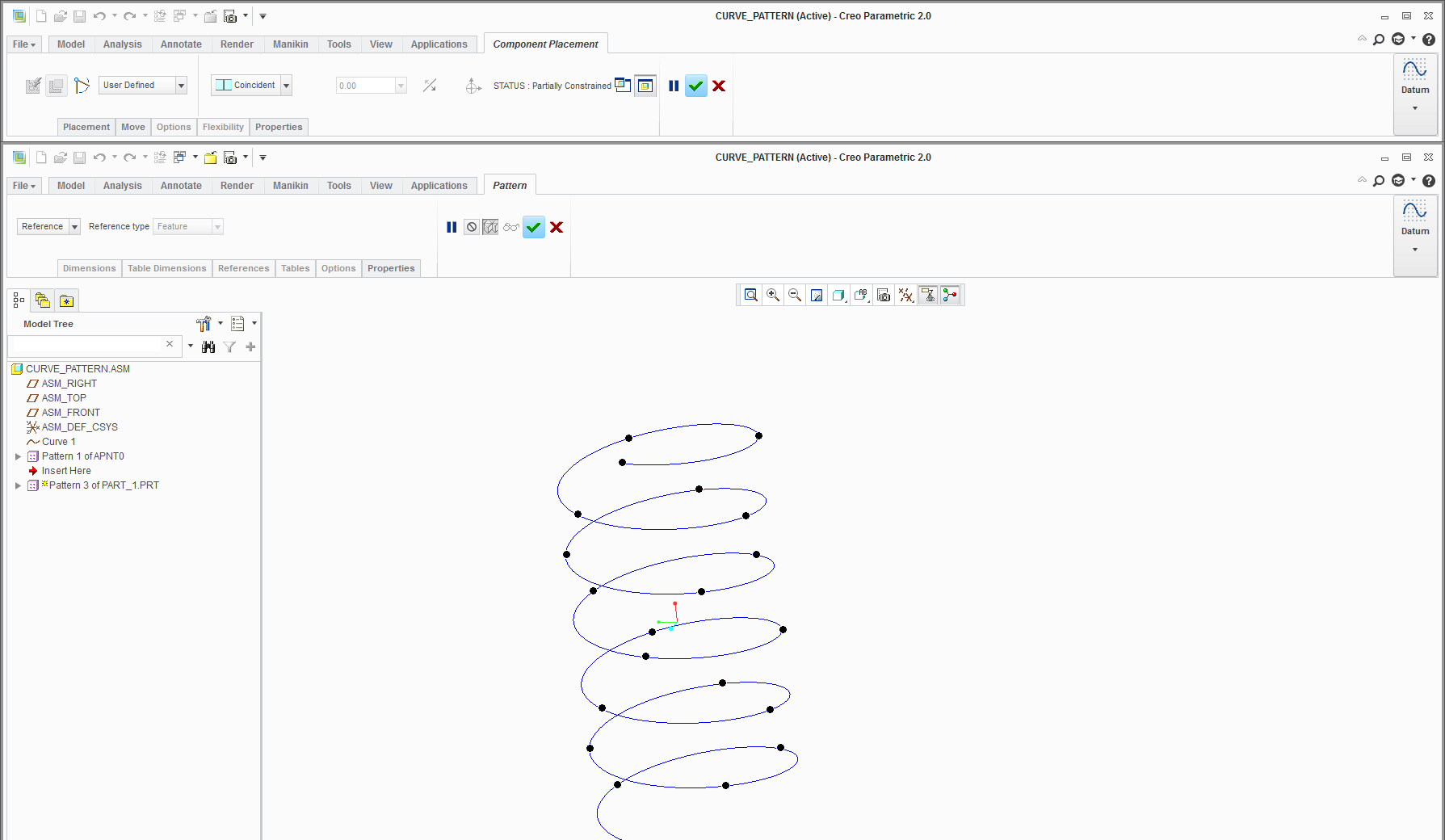# How to pattern linear and circular at the same time?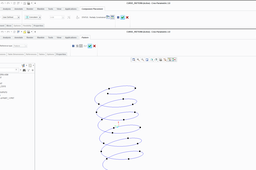This type of pattern could be solved creating a 3D curve (Helix) and patterning datum point on the curve,as I have explained below

1. ### Step 1:

1- Create a sphere of dia 10mm and put a datum point on the center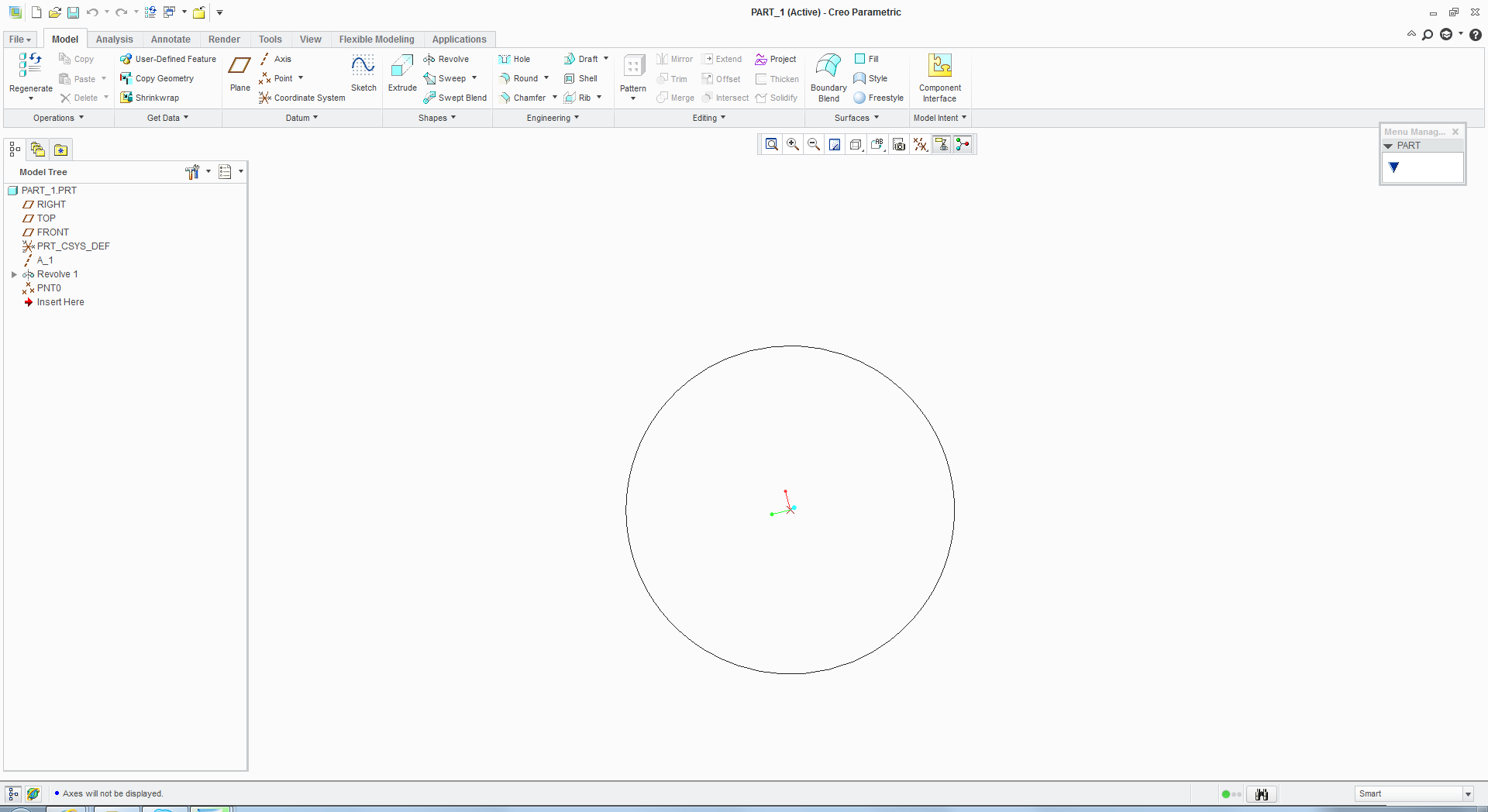2. ### Step 2:

2- Create an assembly , and create a curve using equation
x=200*t
y=50*sin(6*t*360)
z=50*cos(6*t*360)

For a helix with a r=50 and a height of 200

The radius and height varies upon your need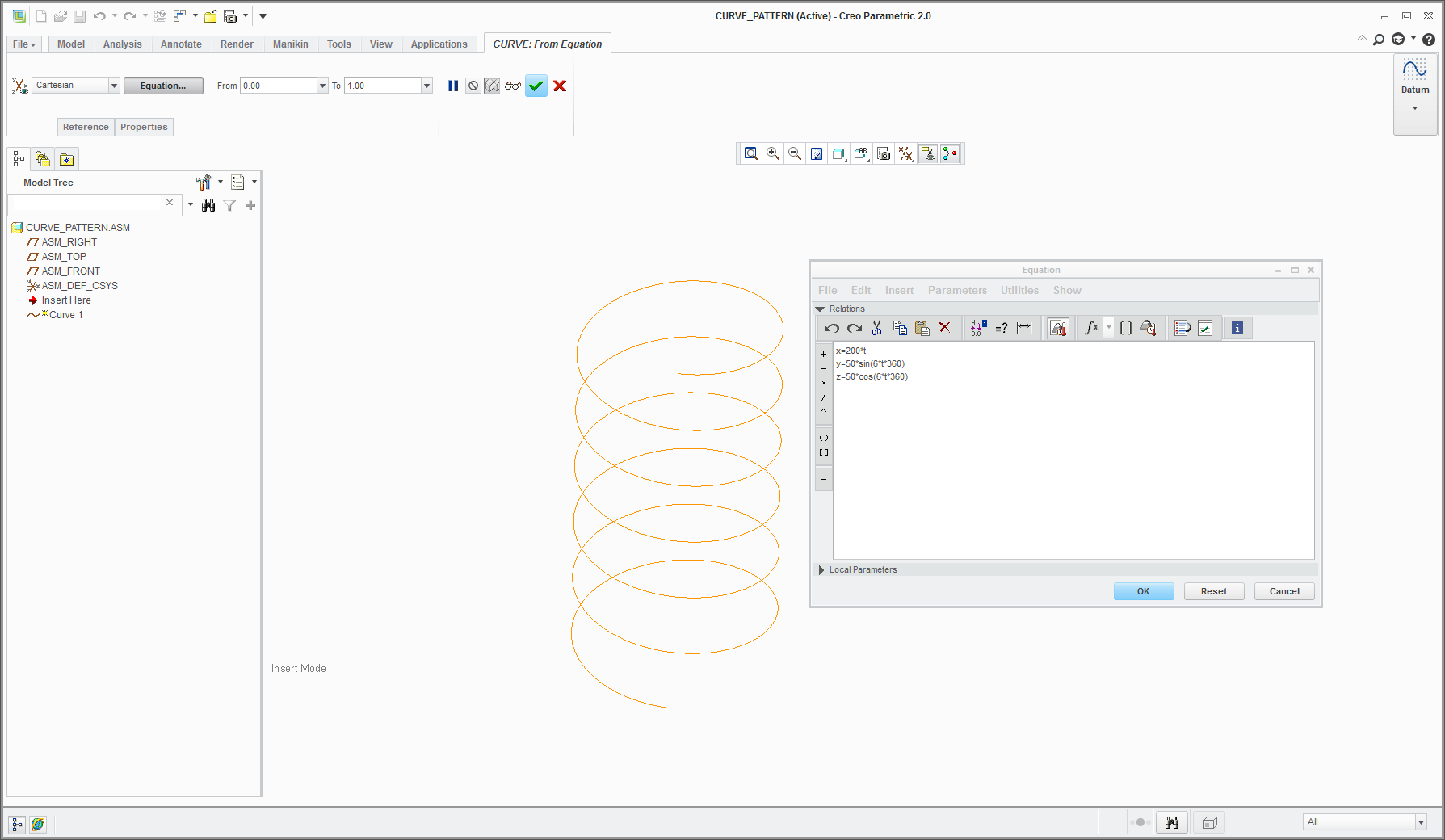3. ### Step 3:

3- Create a datum point on the curve (length ratio =0) , this is the point where you mate/assemble the part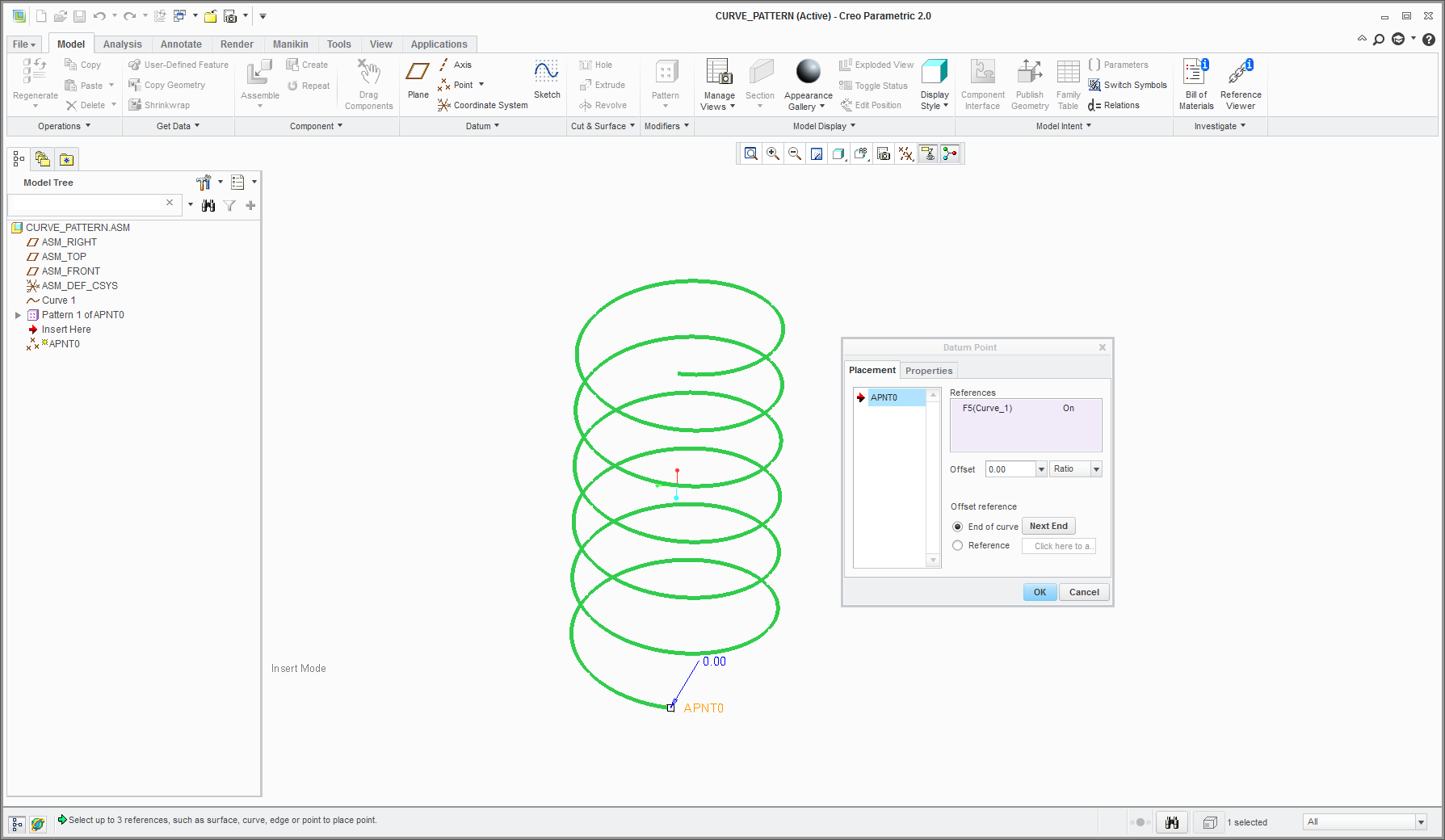4. ### Step 4:

4-Pattern the datum point , using dimension pattern . The no of items depends upon designers descretion, I have choosen 21 items at 0.05 length ratio increment.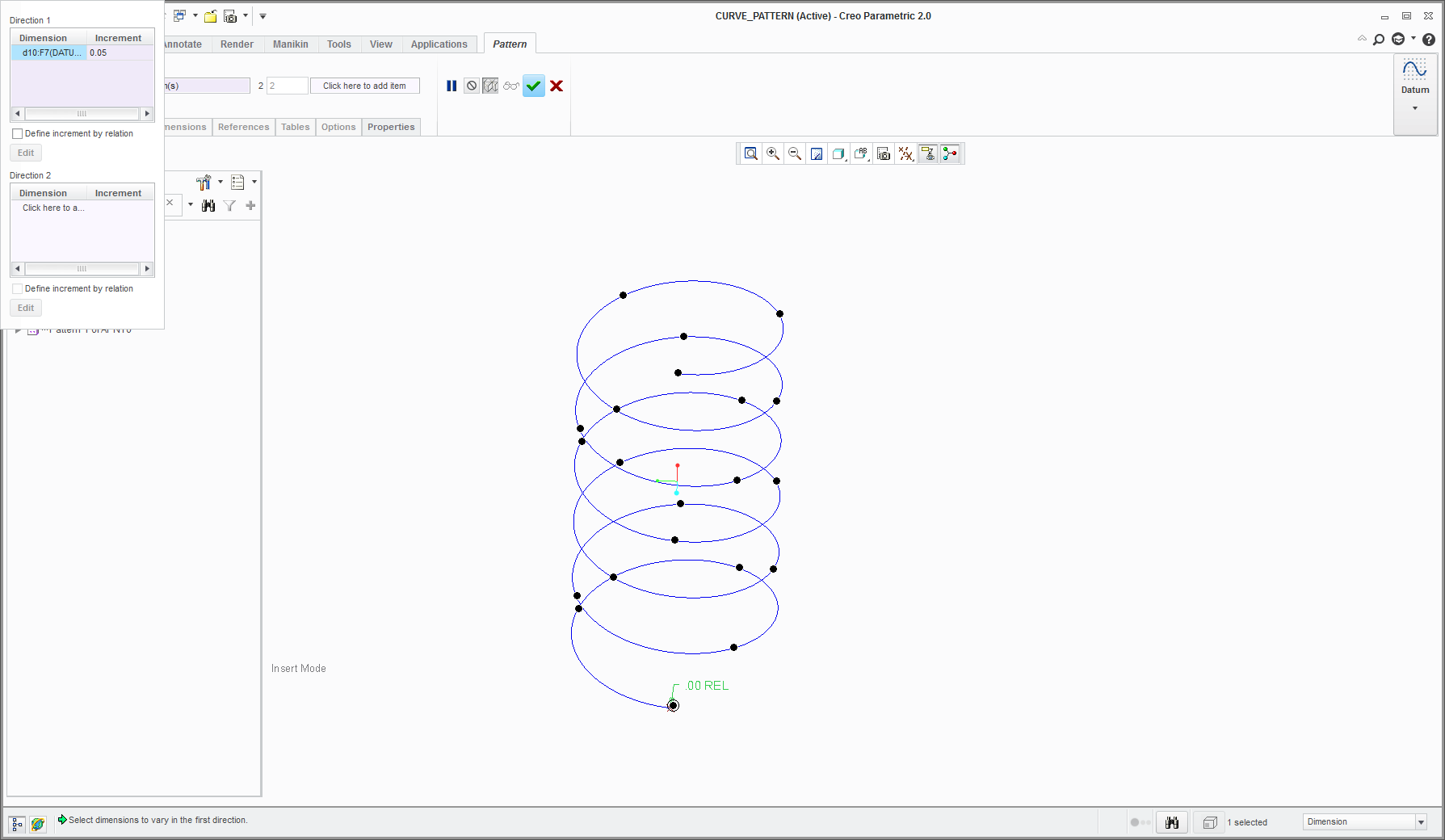5. ### Step 5:

5-Assemble the sphere coinciding the point together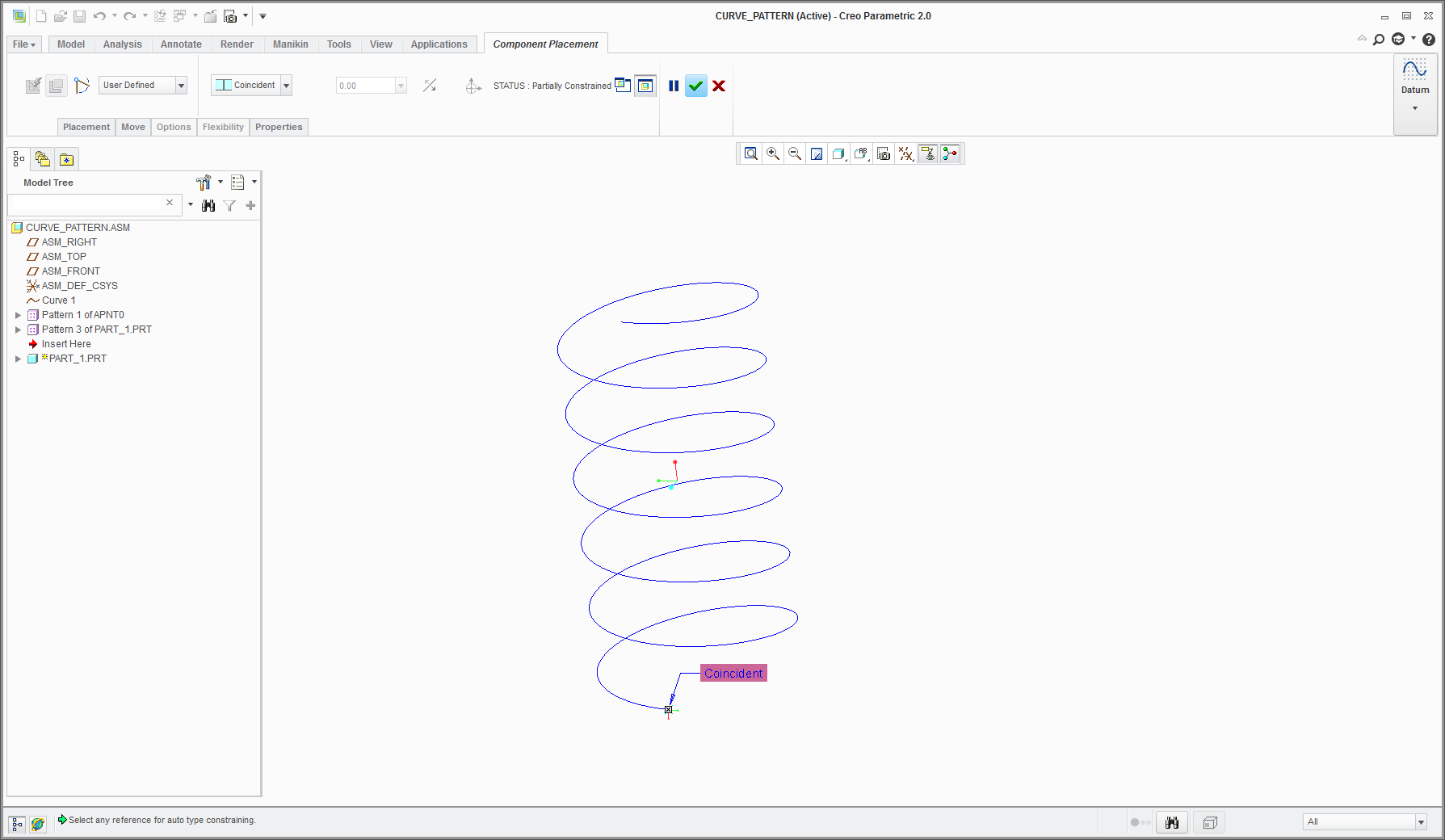6. ### Step 6:

6-Select the part and click the pattern icon, automatically Creo goes for reference pattern .